# 6 Analysis of Multistage Processes

#### DOI

10.34663/9783945561171-08

#### Citation

Arhangel‘skii, Igor V., Dunaev, Alexander V., Makarenko, Irina V., Tikhonov, Nikolay A. and Tarasov, Andrey V. (2013). Analysis of Multistage Processes. In: Non-Isothermal Kinetic Methods: Workbook and Laboratory Manual. Berlin: Max-Planck-Gesellschaft zur Förderung der Wissenschaften.

For multistage process, it is recommended to perform kinetic analysis using the following algorithm. Kinetic analysis is performed separately for each of the stages of the process as for a one-stage reaction, and the corresponding kinetic model and kinetic parameters are determined. Then, kinetic analysis is performed for the entire process using the data obtained for the model of each stage. In so doing, the kinetic parameters of each stage are refined. Thus, when the process is studied as a whole, there is already no need to try different kinetic models since they have been chosen for each stage.

However, for most multistage processes, the effects overlap. To separate quasi-one-stage processes, the Peak Separation software can be used. In general case reaction steps are dependent, so the separation procedure is formal. Obtained peaks does not refer to the single reactions and may be used just for the initial approximation of the Arrenius parameters. In the case of the independent reactions separated peaks refer to the single reactions and thus may be used for calculation of the Arrenius parameters.

## 6.1 Peak Separation Software

In multistage processes with competing or parallel reactions, separate stages, as a rule, overlap, which can lead to considerable errors in the calculated Arrhenius parameters and to an incorrect choice of the scheme of the processes. This in turn will lead to significant errors of the nonlinear regression method because of the nonlinearity of the problem. To solve this problem, the experimental curve is represented by a superposition of separate one-stage processes. This procedure in implemented in the NETZSCH Peak Separation software (Figure 6.1).

The NETZSCH Peak Separation software  fits experimental data by a superposition of separate peaks, each of which can be described by one of the following functions:

1Gaussian function,

2Cauchy function,

3pseudo-Voigt function (the sum of the Gaussian and Cauchy functions with corresponding weights),

4Fraser–Suzuki function (the asymmetric Gaussian function),

5Pearson function (monotonic transformation from the Gaussian to the Cauchy function),

6modified Laplace function.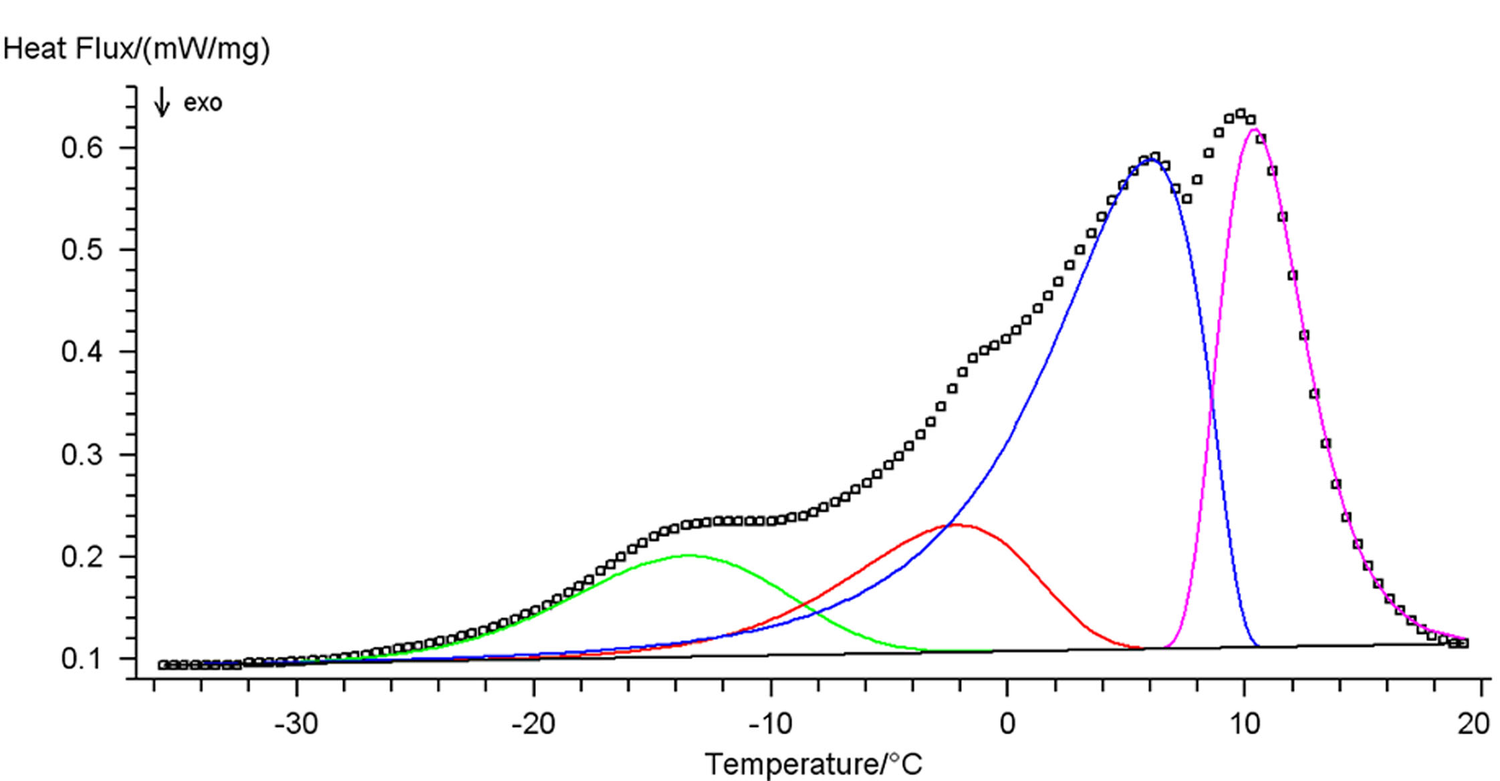Fig. 6.1: Peak separation using the Peak Separation software (reprinted with permission from  © NETZSCH-Gerätebau GmbH).

Fig. 6.1: Peak separation using the Peak Separation software (reprinted with permission from  © NETZSCH-Gerätebau GmbH).

In thermal analysis, chemical reaction steps are described in most cases by the Fraser–Suzuki function (asymmetric Gaussian function). For other processes, e.g., polymer melting, the modified Laplace function must be used. Figure 6.1 shows the decomposition of a multimodal curve with the use of this function and its analytical representation. The Fraser–Suzuki function (asymmetric Gaussian function) and its graphical representation are given below.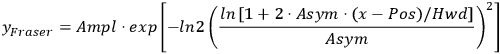6.1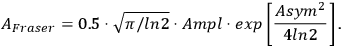6.2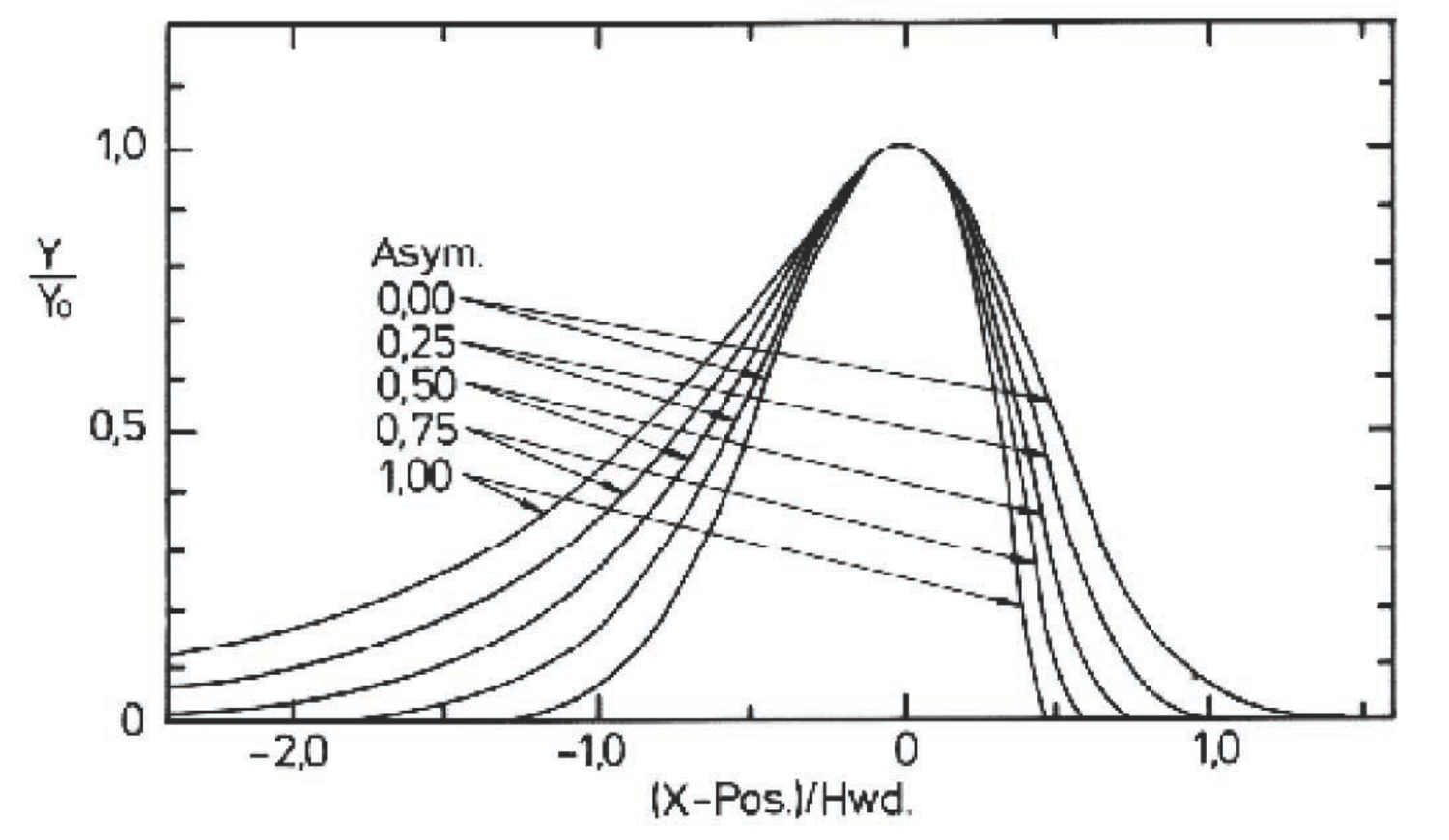Fig. 6.2: Graph of the Fraser–Suzuki function (reprinted with permission from  © NETZSCH-Gerätebau GmbH).

Fig. 6.2: Graph of the Fraser–Suzuki function (reprinted with permission from  © NETZSCH-Gerätebau GmbH).

The software outputs the following parameters:

1the optimal curve parameters and their standard deviations,

2statistical parameters characterizing the fit quality (correlation coefficient and so on.),

3the initial and simulated curves with the graphs of separate peaks,

4calculated areas under separate peaks and their contribution to the total area under the curve.

## 6.2 Multiple Step Reaction Analysis as Exemplified by the Carbonization of Oxidized PAN Fiber

First, multimodal curves are decomposed into separate components and parameters of each process are found by the above procedures, assuming that all processes are quasi-one-stage reactions. Then, the phenomenon is described as a whole. For multiple step processes, the program suggests a list of some schemes of similar transformations, and appropriate schemes are selected from this list. The schemes are presented in Figure 1 of the Appendix. The choice of the corresponding scheme is based on some a priori ideas about the character of stages of the process under consideration.

As an example of the kinetic analysis of multistage processes, let us consider the carbonization of oxidized polyacrylonitrile fiber yielding carbon fiber. The results of thermogravimetric analysis are convenient to use as input data (Figure 6.3).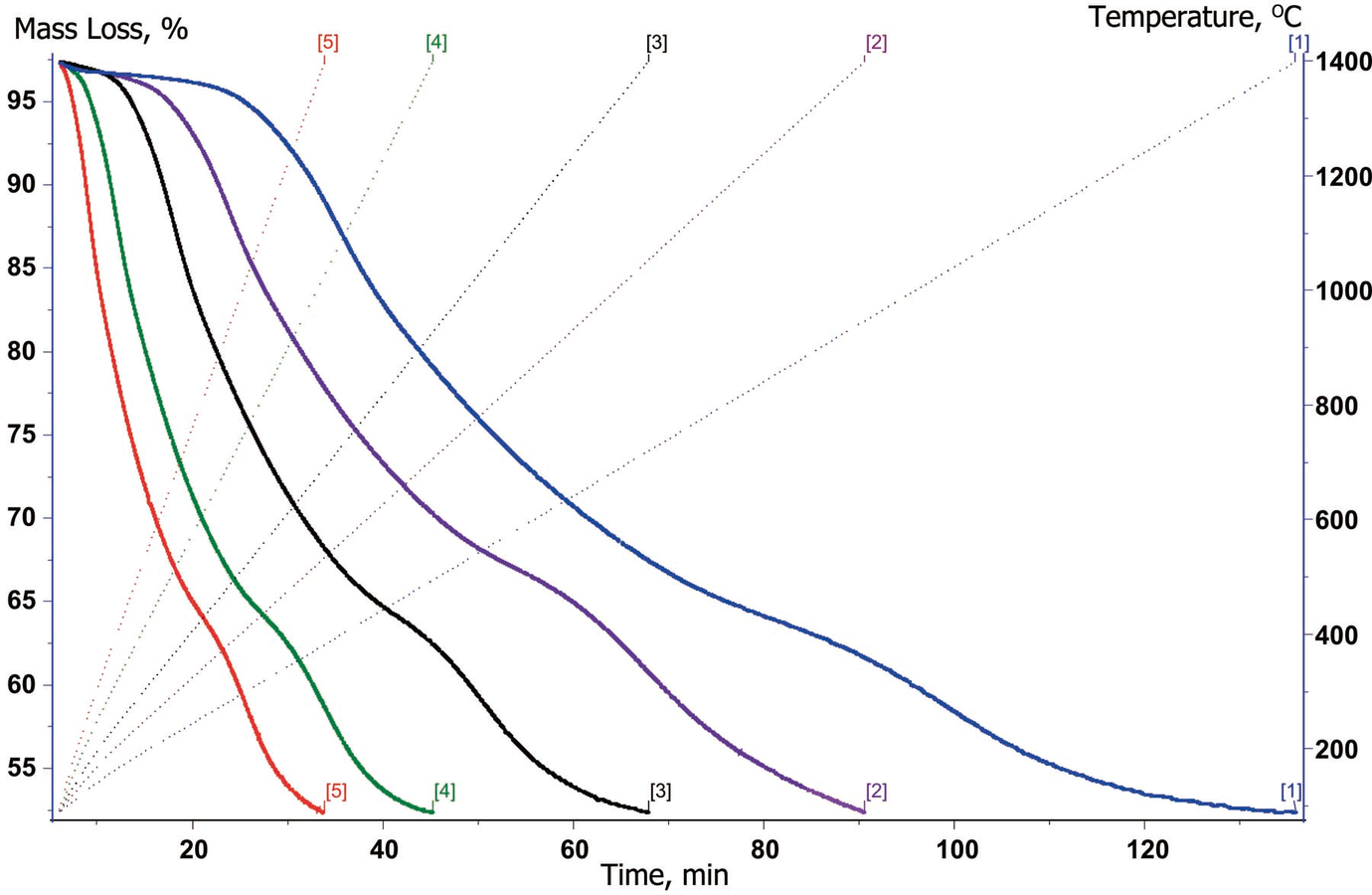Fig. 6.3: Initial TG weight loss curves recorded at different heating rates: (1) 10, (2) 15, (3) 20, (4) 30, and (5) 40 K/min.

Fig. 6.3: Initial TG weight loss curves recorded at different heating rates: (1) 10, (2) 15, (3) 20, (4) 30, and (5) 40 K/min.

The carbonization is a complex process and occurs in several stages [14,15], which is clearly reflected by the presence of several DTG peaks (Figure 6.4). Kinetic description of a multistage process starts with the decomposition of the complex DTG profile into separate components. It is assumed that the carbonization is, at least, a four-stage process [14,15]. To separate different stages, the initial DTG curves should be loaded into the Peak Separation software, which provides the best-fit description of the initial process by varying the position, asymmetry, and width of discrete peaks (Figure 6.5) at each heating rate.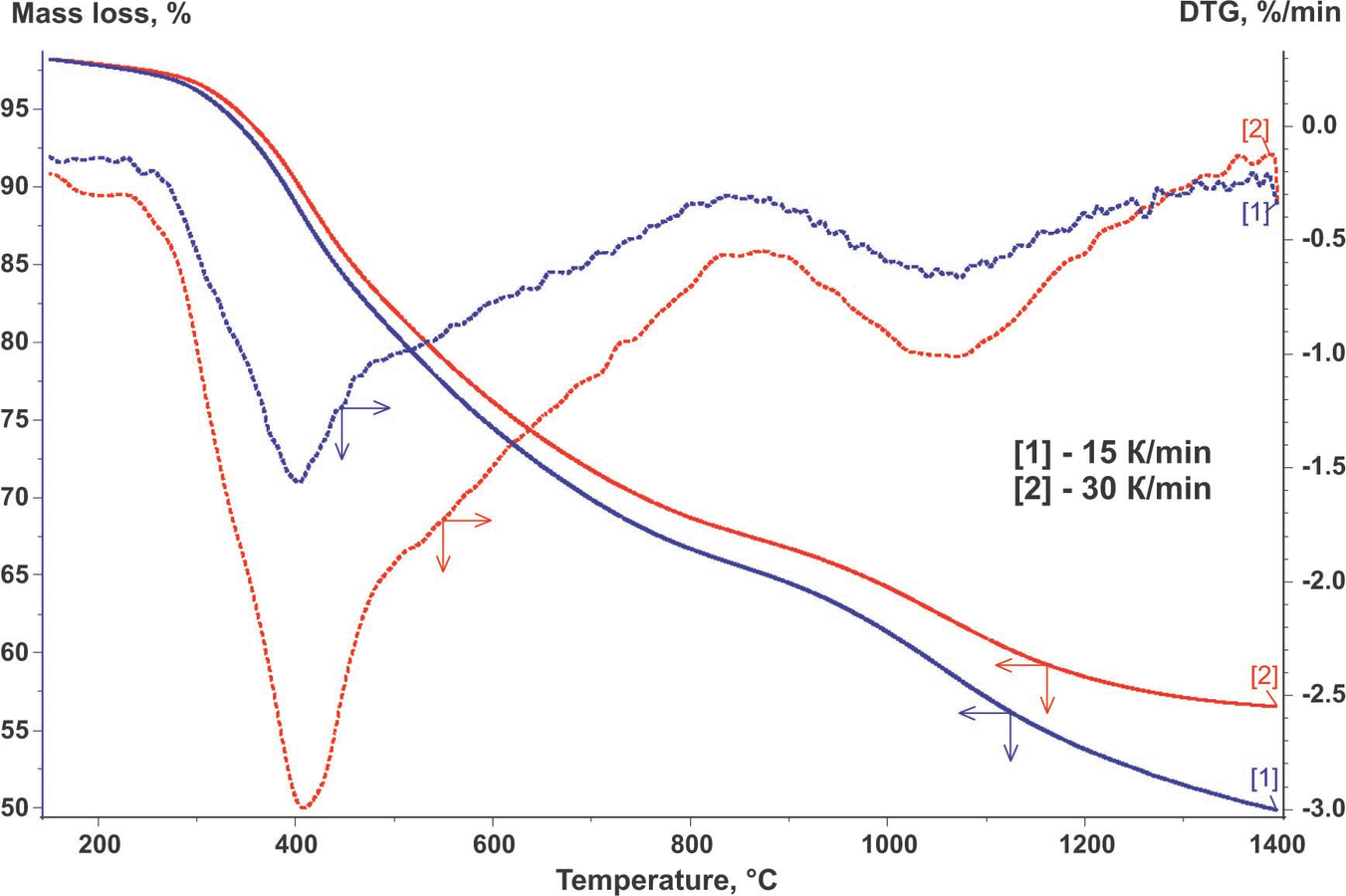Fig. 6.4: DTG curves obtained by differentiation of the initial TG curves recorded at (1) 15 and (2) 30 K/min.

Fig. 6.4: DTG curves obtained by differentiation of the initial TG curves recorded at (1) 15 and (2) 30 K/min.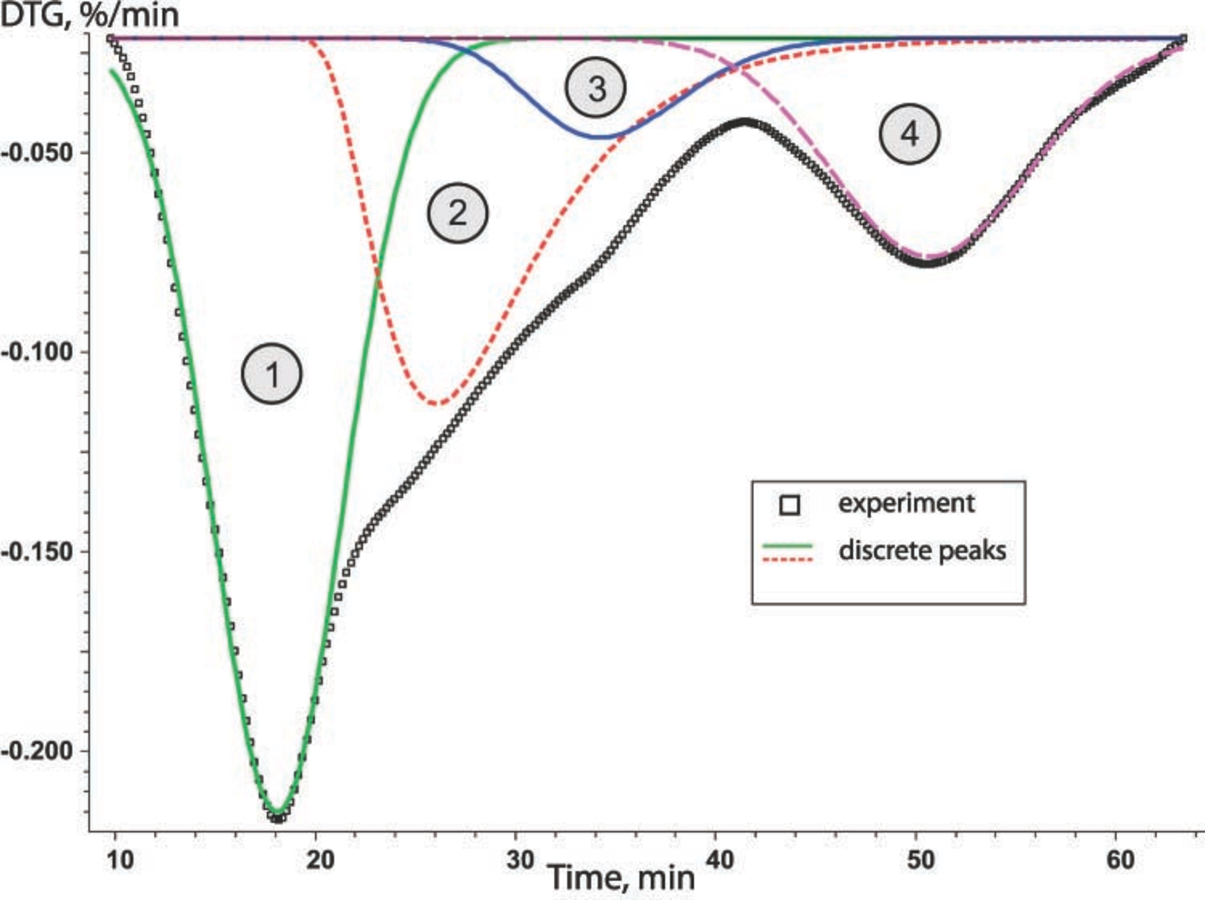Fig. 6.5: Decomposition of the overall DTG profile (10 K/min) into components with the Peak Separation software.

Fig. 6.5: Decomposition of the overall DTG profile (10 K/min) into components with the Peak Separation software.

Then, the resulting peaks are sorted on the basis of the number of stage and the inverse kinetic problem is solved. As a result, we obtain the type of model for the given stage and approximate Arrhenius parameters. As a rule, several models of comparable statistical significance satisfy the solution of this problem. If no information is available on the true mechanism of the process, both variants are used for solving the direct kinetic problem.

The estimated parameters and types of models calculated at this step of calculations are listed in Table 6.1, and statistical data of the calculation is presented in Table 6.3. In Table 6.1, the “apparent” activation energy is expressed in kelvins since the notion of mole has no physical meaning for fiber. The Ea/R constant is the temperature coefficient of the reaction rate.

N Kinetic model Ea/R*103K t•S log A t•S n t•S
1 Avrami–Erofeev equation 25.0 0.6 14.8 0.4 0.28 0.3 x 10-3
2 nth order equation 17.2 0.8 7.2 6.5 3.0 0.13
3 Avrami–Erofeev equation 41.1 2.3 16.2 1.0 0.29 0.1 x 10-3
4 Avrami–Erofeev equation 54.8 1.3 16.0 0.4 0.32 3.3 x 10-3

Tab. 6.1: Estimated kinetic models and parameters of separate stages of the carbonization process.

Tab. 6.1: Estimated kinetic models and parameters of separate stages of the carbonization process.

N Kinetic model EaR*103K t•S log A t•S n t•S
1 Avrami–Erofeev equation 26.1 0.6 15.7 0.4 0.25 4.2*10-3
2 nth order equation 35.5 2.1 18.8 1.2 1.98 0.14
3 Avrami–Erofeev equation 38.3 2.1 16.7 1.0 0.28 0.1*10-3
4 Avrami–Erofeev equation 56.8 4.7 16.8 1.6 0.19 0.1*10-3

Tab. 6.2: Calculated kinetic models and parameters of separate stages of the carbonization process.

Tab. 6.2: Calculated kinetic models and parameters of separate stages of the carbonization process.

Parameter Stage 1 Stage 2 Stage 3 Stage 4
Least-squares value 22.3 32.0 2.6 7.1
Correlation coefficient 0.9988 0.9926 0.9956 0.9979
Average difference 2.2 x 10-3 21.0 x 10-3 8.6 x 10-3 14.5 x 10-3
Durbin–Watson test value 2.8 x 10-3 1.4 x 10-3 1.6 x 10-3 1.4 x 10-3
Durbin–Watson ratio 19.0 26.9 25.0 27.0

Tab. 6.3: Statistical data of the calculation.

Tab. 6.3: Statistical data of the calculation.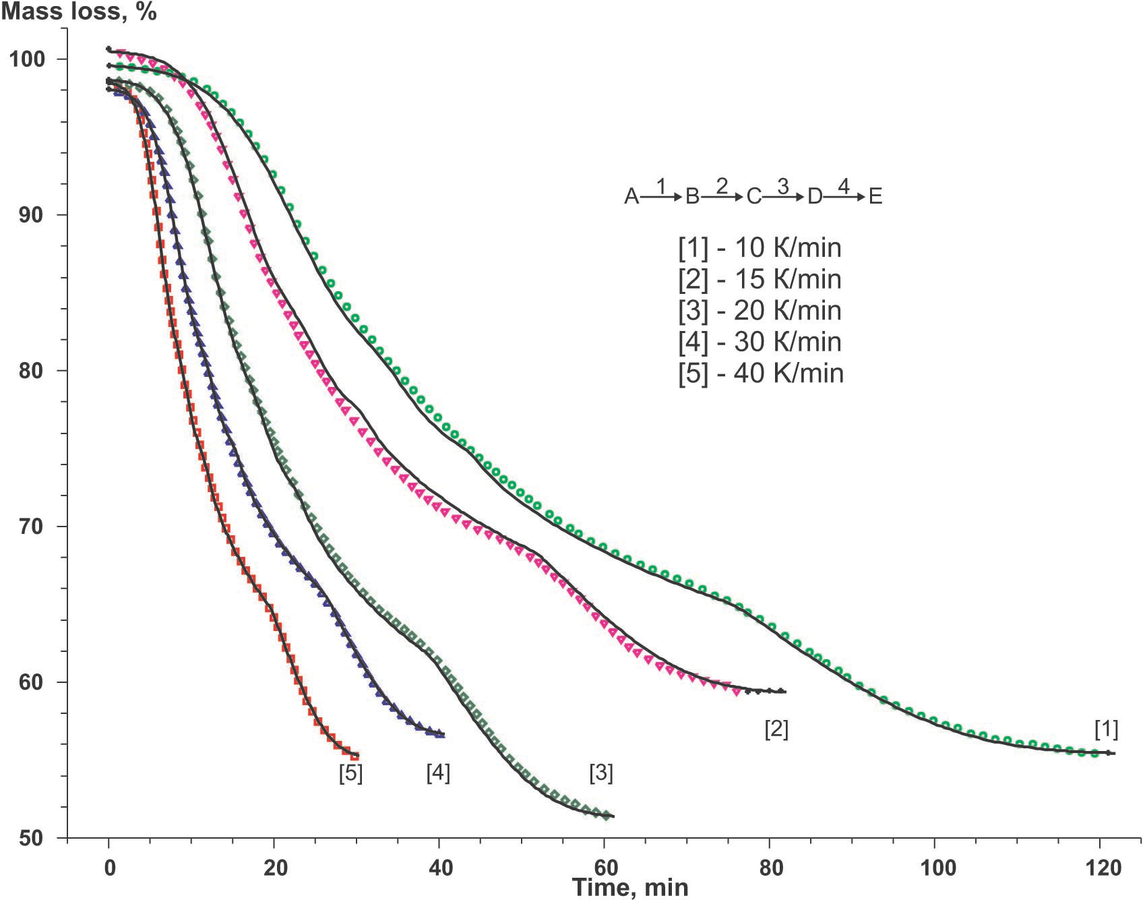Fig. 6.6: Complete kinetic description of experimental data with the use of the model of four successive reactions.

Fig. 6.6: Complete kinetic description of experimental data with the use of the model of four successive reactions.

One of the basic tasks of formal kinetic description is to determine the sequence of stages. First, the direct kinetic problem is solved using multivariate nonlinear regression for the successive processes (the qfff scheme in Figure 2 of Appendix, four-stage). Then, for each of the stages, kinetic parameters are refined separately on the basis of statistical tests. As shown by preliminary calculations, stages 1, 3, and 4 are well fitted by the Avrami–Erofeev equation (Equation 3.5) and stage 2, by the nth order equation.6.3

The calculated results and experimental data for successive processes are compared in Figure 6.6. The kinetic parameters and statistical characteristics of this sequence of stages are presented in Tables 6.4 and 6.5.

Parameter Value
Least-squares value 1448.7
Correlation coefficient 0.9998
Average difference 0.21
Durbin–Watson test value 5.09 x 10-3
Durbin–Waton ratio 14.4

Tab. 6.4: Statistical data of the calculation.

Tab. 6.4: Statistical data of the calculation.

N Kinetic model EaR*103K t•S log A t•S n t•S
1 Avrami–Erofeev equation 24 3 11 1.5 0.23 1.2 x 10-2
2 nth order equation 34 7 10 3 5 1
3 Avrami–Erofeev equation 63 5 25 5 0.02 0.1
4 Avrami–Erofeev equation 99 230 53 116 0.8 10

Tab. 6.5: Calculated kinetic models and parameters of separate stages of the carbonization process for the successive-parallel scheme.

Tab. 6.5: Calculated kinetic models and parameters of separate stages of the carbonization process for the successive-parallel scheme.

According to the authors  carbonization process may be represented by a set of successive-parallel processes. Let us consider this situation (the qffc scheme in Figure 3 of the Appendix). The results shown in Figure 6.3 and summarized in Table 6.5 have been obtained with the use of the same set of functions.

The F test value for the latter scheme with respect to the former one is

Fexp = 58.6, Ftheor = 1.11.

Comparison of the calculation results for each scheme reliably shows that, from the formal kinetic viewpoint, the scheme of successive stages best describes thermoanalytical data as a whole.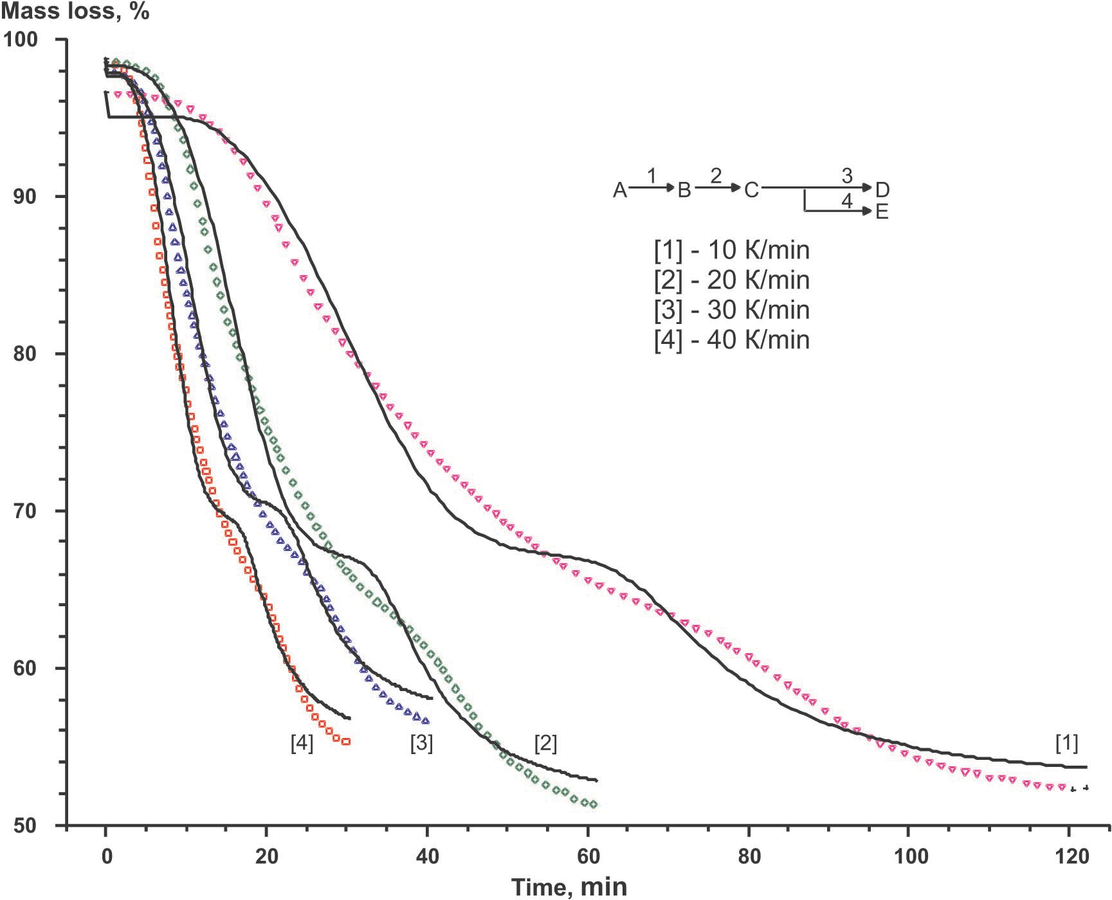Fig. 6.7: Comparison of calculated and experimental data for the scheme of successive-parallel stages.

Fig. 6.7: Comparison of calculated and experimental data for the scheme of successive-parallel stages.

Thus, formal kinetic analysis makes it possible to choose the type of function best fitting the experimental curves (TG, DTG, DSC), evaluate kinetic parameters, determine the number of stages and their sequence (successive, parallel, etc.). In addition, the statistically optimal kinetic parameters allow one to model temperature change conditions resulting in constant weight loss or enthalpy change rate and obtain dependences of these characteristics under isothermal conditions or in other temperature programs.

#### 6.2.1 References

 J. Sestak. Thermophysical Properties of Solids. Prague: Academia, 1984

 S. Vyazovkin al.. ICTAC Kinetics Committee Recommendations for Performing Kinetic Computations on Thermal Analysis Data Thermochimica Acta 1: 520 (2011): 520.

 V.A. Sipachev, I.V. Arkhangel ' skii. Calculation Techniques Solving Non-Isothermal Kinetic Problems Journal of Thermal Analysis 38: 1283-1291 (1992): 1283-1291.

 B. Delmon. Introduction to Heterogeneous Kinetics. Paris: Technip, 1969

 P. Barret. Kinetics in Heterogeneous Chemical Systems., 1974

 H.L. Friedman. A Quick, Direct Method for the Determination of Activation Energy from Thermogravimetric Data J. Polym. Lett. 4(5): 323-328 (1966): 323-328.

 T. Ozawa. A New Method of Analyzing Thermogravimetric Data Bull. Chem. Soc. Jpn. 38: 1881-1886 (1965): 1881-1886.

 J.H. Flynn, L.A. Wall. A Quick, Direct Method for the Determination of Activation Energy from Thermogravimetric Data J. Polym. Sci. Polym. Lett. 4: 323-328 (1966): 323-328.

 NETZSCH-Thermokinetics 3.1 Software Help. NETZSCH-Thermokinetics 3.1 Software Help. .

 J. Opfermann. Kinetic Analysis Using Multivariate Non-Linear Regression Journal of Thermal Analysis & Calorimetry 60: 641-658 (2000): 641-658.

 S.Z.D. Cheng. Handbook of Thermal Analysis and Calorimetry. Applications to Polymers and Plastics., 2001

 L. Shechter, J. Wynstra, J. W.. Glycidyl Ether Reactions with Amines Ind. Eng. Chem. 48: 94-97 (1956): 94-97.

 NETZSCH-Peakseparation 3.0 Software Help.. NETZSCH-Peakseparation 3.0 Software Help.. .

 P. Morgan. Carbon Fibers and Their Composites. New York: TaylorFrancis, 2005

 V.Y. Varshavskii. Carbon Fibers. Moscow: Varshavskii, 2005